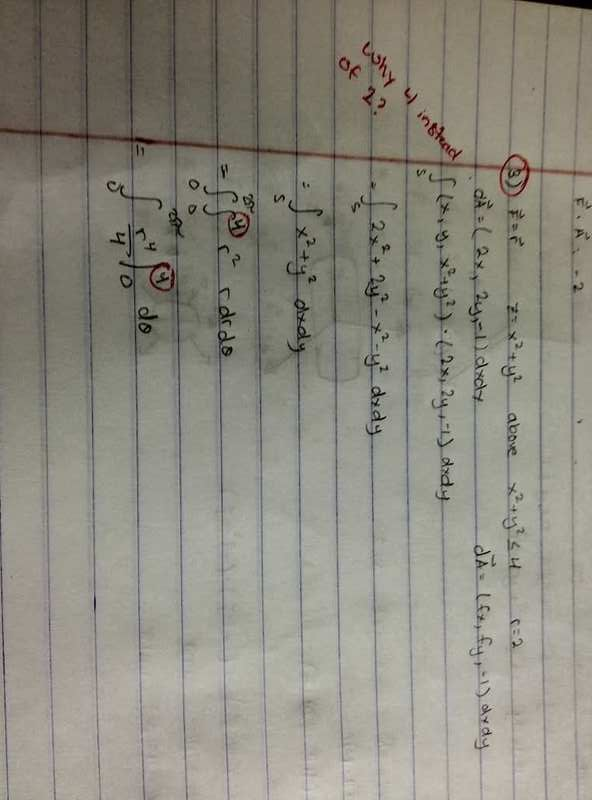# Calculation Flux integral

QUESTION: Compute the flux of the vector field, F , through the surface, S.

F = xi+yj+zk

S is the part of the surface z = x^2 + y^2 above the disc x^2 + y^2 ≤ 4 , oriented downward.

I am just wondering why the integrand is from o to 4 while the radius is only 2.HallsofIvy